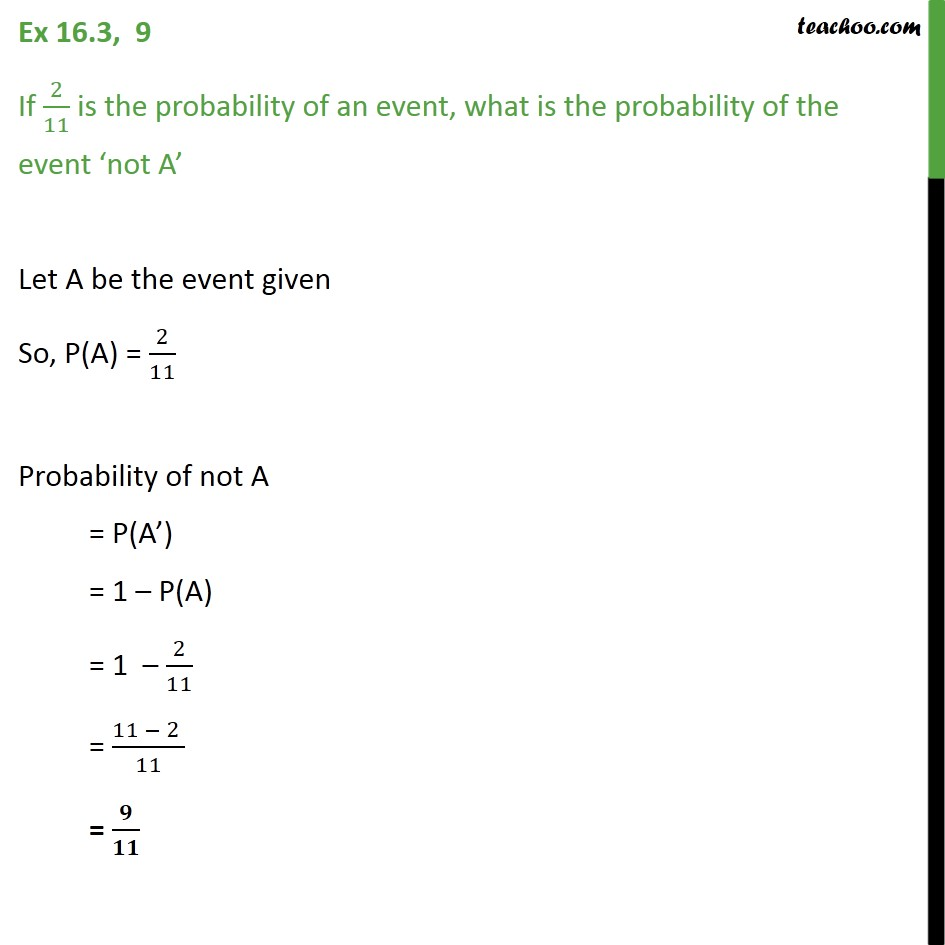Ex 16.3

Chapter 16 Class 11 Probability
Serial order wiseGet live Maths 1-on-1 Classs - Class 6 to 12

### Transcript

Ex 16.3, 9 If 2 11 is the probability of an event, what is the probability of the event not A Let A be the event given So, P(A) = 2 11 Probability of not A = P(A ) = 1 P(A) = 1 2 11 = 11 2 11 =